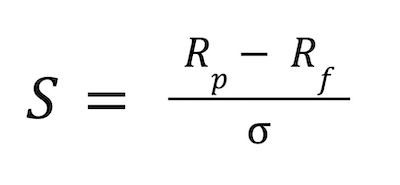## Module 6

### What is unsystematic risk?

Sharpe Ratio## Sharpe Ratio

The Sharpe ratio calculates the return that an investor will receive for an investment, compared to its risk. It allows investors to quickly identify the risk profile of individual stocks.

The method can not only be used with stocks but can be used to measure the return of a portfolio, in comparison to the risk associated with it.

A more diversified portfolio will have a higher Sharpe ratio than a portfolio that is less diversified. That is the case because diversification reduces the amount of risk that an investor is exposed to, in relation to their return.

The Sharpe ratio can be used with historic information or estimates of expected future performance.

## Calculating the Sharpe ratio

In order to calculate the Sharpe ratio, the following formula is used.Everything that has been included in this formula has been covered previously in this course. Rp refers to the average return of a portfolio (or stock), Rf refers to the risk-free rate and σ refers to standard deviation of the portfolio (or stock).

Therefore, the Sharpe ratio is calculated by subtracting the risk-free rate from the average return of a portfolio (or stock). The sum of that calculation is then divided by the standard deviation of that portfolio (or stock), to give you the Sharpe ratio.

For example, if the risk-free rate is 1%, the return of a stock is 15% and its standard deviation is 12%, then the Sharpe ratio would be: (15% – 1%) ÷ 12% = 1.167.

A Sharpe ratio of 1 is considered good in terms of the return of the investment in relation to amount of risk taken, while a Sharpe ratio of 2 or above is considered very good and a Sharpe Ratio of 3 or above is considered outstanding.

## Drawbacks of the Sharpe ratio

Although Sharpe ratios have their merits, it is probably not as easy to use or understand as beta. This is especially true if you are new to investing and have little experience.

A major flaw of the Sharpe ratio is that it uses standard deviation and the average return of an investment, which changes constantly in reality. Investments very rarely follow their average return and are not normally distributed.

Another issue with the Sharpe ratio is that results vary dramatically, depending on the timeframe taken by the investor. If an investor decides to measures the average return based on daily returns, this will lead to a lot more volatility than monthly returns.

Finally, as with other measures, the Sharpe ratio relies mostly on historic information, which may not provide a fair indication of what is going to happen in the future.